Open in App
Not now

# Implementing interactive Online Shopping in C++

• Difficulty Level : Hard
• Last Updated : 07 Jul, 2021

Online shopping is all about calculating the total amount for the items selected by the customer. In this article, we will discuss a menu-driven C++ program for Online Shopping.

### Functionality Provided

1. Users will be able to purchase laptops, Mobile, Computer Courses.
2. Users will be able to add items.
3. Users will be able to reduce the quantity or delete the item.
4. Users will be able to print the bill.

### Approach

Firstly a menu will be displayed to the customer. After the selection, all the products with their prices will be displayed. Then the customer will select the products and chooses the quantity(number of products). This process continues until the shopping is completed. Whenever the customer completes his shopping, the items, quantity, cost, and finally the total amount to be paid are displayed.

Below is the implementation of the above functionality:

## C++

 `// C++ program to implement the program``// that illustrates Online shopping``#include ``#include ``#include ``#include ``using` `namespace` `std;` `char` `c1, confirm_quantity;``float` `quantity;``int` `selectedNum;``double` `total_amount = 0;``int` `flag = 0;` `// Stores items with their corresponding``// price``map items = {``    ``{ ``"Samsung"``, 15000 },``    ``{ ``"Redmi"``, 12000 },``    ``{ ``"Apple"``, 100000 },``    ``{ ``"Macbook"``, 250000 },``    ``{ ``"HP"``, 40000 },``    ``{ ``"Lenovo"``, 35000 },``    ``{ ``"C"``, 1000 },``    ``{ ``"C++"``, 3000 },``    ``{ ``"Java"``, 4000 },``    ``{ ``"Python"``, 3500 }``};` `// Stores the selected items with``// their quantity``map selected_items;` `// Function to print the bill after shopping``// is completed prints the items, quantity,``// their cost along with total amount``void` `printBill(map items,``               ``map selected_items,``               ``float` `total_amount)``{``    ``cout << ``"Item      "``         ``<< ``"Quantity      "``         ``<< ``"Cost\n"``;` `    ``for` `(``auto` `j = selected_items.begin();``         ``j != selected_items.end(); j++) {``        ``cout << j->first << ``"        "``;``        ``cout << j->second << ``"          "``;``        ``cout << (selected_items[j->first])``                    ``* (items[j->first])``             ``<< endl;``    ``}` `    ``cout << ``"-----------------------"``         ``<< ``"-------------\n"``;``    ``cout << ``"Total amount:             "``         ``<< total_amount << endl;``    ``cout << ``"-----------------------"``         ``<< ``"-------------\n"``;``    ``cout << ``"*****THANK YOU && HAPPY"``         ``<< ``" ONLINE SHOPPING*****"``;``}` `// Function to ask the basic details of``// any customer``void` `customerDetails()``{` `    ``cout << ``"Enter your name: "``;``    ``string customer_name;``    ``getline(cin, customer_name);` `    ``cout << ``"WELCOME "``;``    ``for` `(``int` `i = 0;``         ``i < customer_name.length();``         ``i++) {``        ``cout << ``char``(``toupper``(``            ``customer_name[i]));``    ``}``    ``cout << ``"\n"``;``}` `// showMenu() is to print the``// menu to the user``void` `showMenu()``{``    ``cout << ``"Menu\n"``;``    ``cout << ``"=  =  =  =  =  =  =  = "``         ``<< ``" =  =  =  =  = \n"``;``    ``cout << ``"1.Mobile\n2.laptop\n3"``         ``<< ``".Computer courses\n"``;``    ``cout << ``"=  =  =  =  =  =  =  = "``         ``<< ``" =  =  =  =  = \n"``;``}` `// Function to display the mobile products``void` `showMobileMenu()``{``    ``cout << ``"- - - - - - - - - - -"``         ``<< ``" - -\nItem       Cost\n"``;``    ``cout << ``"1.Samsung  Rs.15, 000/-\n"``;``    ``cout << ``"2.Redmi    Rs.12, 000/-\n"``;``    ``cout << ``"3.Apple    Rs.1, 00, 000/-\n"``;``    ``cout << ``"- - - - - - - - - - - - -\n"``;``}` `// Function to display Laptop products``void` `showLaptopMenu()``{``    ``cout << ``"- - - - - - - - - - -"``         ``<< ``" - -\nItem       Cost\n"``;``    ``cout << ``"1.Macbook  Rs.2, 00, 000/-\n"``;``    ``cout << ``"2.HP       Rs.40, 000/-\n"``;``    ``cout << ``"3.Lenovo   Rs.35, 000/-\n"``;``    ``cout << ``"- - - - - - - - - - - - -\n"``;``}` `// if the user selects computer courses,``// then courses list will be displayed``void` `showComputerCourseMenu()``{``    ``cout << ``"- - - - - - - - - - "``         ``<< ``" - -\nItem       Cost\n"``;``    ``cout << ``"1.C        Rs.1, 000/-\n"``;``    ``cout << ``"2.C++      Rs.3, 000/-\n"``;``    ``cout << ``"3.Java     Rs.4, 000/-\n"``;``    ``cout << ``"4.Python   Rs.3, 500/-\n"``;``    ``cout << ``"- - - - - - - - - - - - -\n"``;``}` `// Function to display the mobile category``void` `selectedMobile()``{``    ``cout << ``"Do you wish to conti"``         ``<< ``"nue?(for yes"` `+ ``"press (Y/y ), "``         ``<< ``" if no press other letter ): "``;``    ``cin >> c1;` `    ``if` `(c1 == ``'Y'` `|| c1 == ``'y'``) {``        ``cout << ``"Enter respective number: "``;``        ``cin >> selectedNum;` `        ``if` `(selectedNum == 1``            ``|| selectedNum == 2``            ``|| selectedNum == 3) {` `            ``// Selected Samsung``            ``if` `(selectedNum == 1) {` `                ``cout << ``"selected Samsung\n"``;``                ``do` `{``                    ``cout << ``"Quantity: "``;` `                    ``cin >> quantity;` `                    ``cout << ``"You have selected Samsung - "``                         ``<< quantity << endl;``                    ``cout << ``"Are you sure?"``                         ``<< ``"(for yes press (Y/y ), "``                         ``<< ``" if no press other letter): "``;` `                    ``cin >> confirm_quantity;` `                ``} ``while` `((confirm_quantity != ``'y'``                          ``&& confirm_quantity != ``'Y'``)``                         ``|| (quantity < 0)``                         ``|| (``ceil``(quantity) != ``floor``(quantity)));` `                ``if` `(confirm_quantity == ``'y'``                    ``|| confirm_quantity == ``'Y'``) {``                    ``total_amount += quantity``                                    ``* items[``"Samsung"``];``                    ``selected_items[``"Samsung"``] = quantity;``                    ``cout << ``"amount  =  "``                         ``<< total_amount << endl;``                ``}``            ``}` `            ``// Selected Redmi``            ``if` `(selectedNum == 2) {` `                ``cout << ``"selected Redmi\n"``;` `                ``do` `{``                    ``cout << ``"Quantity: "``;``                    ``cin >> quantity;``                    ``cout << ``"You have selec"``                         ``<< ``"ted Redmi - "``                         ``<< quantity << endl;``                    ``cout << ``"Are you sure?(f"``                         ``<< ``"or yes press (Y/y ), "``                         ``<< ``" if no press other letter ): "``;``                    ``cin >> confirm_quantity;``                ``} ``while` `((confirm_quantity != ``'y'``                          ``&& confirm_quantity != ``'Y'``)``                         ``|| (quantity < 0)``                         ``|| (``ceil``(quantity)``                             ``!= ``floor``(quantity)));` `                ``if` `(confirm_quantity == ``'y'``                    ``|| confirm_quantity == ``'Y'``) {` `                    ``total_amount += quantity``                                    ``* items[``"Redmi"``];``                    ``selected_items[``"Redmi"``] = quantity;``                    ``cout << ``"amount  =  "``                         ``<< total_amount << endl;``                ``}``            ``}` `            ``// Selected Apple``            ``if` `(selectedNum == 3) {` `                ``cout << ``"You have selected Apple\n"``;` `                ``do` `{``                    ``cout << ``"Quantity: "``;``                    ``cin >> quantity;``                    ``cout << ``"You have selected"``                         ``<< ``" Apple - "``                         ``<< quantity``                         ``<< endl;``                    ``cout << ``"Are you sure?"``                         ``<< ``"(for yes press (Y/y )"``                         ``<< ``", if no press other letter ): "``;``                    ``cin >> confirm_quantity;``                ``} ``while` `((confirm_quantity != ``'y'``                          ``&& confirm_quantity != ``'Y'``)``                         ``|| (quantity < 0)``                         ``|| (``ceil``(quantity)``                             ``!= ``floor``(quantity)));` `                ``if` `(confirm_quantity == ``'y'``                    ``|| confirm_quantity == ``'Y'``) {``                    ``total_amount += quantity``                                    ``* items[``"Apple"``];``                    ``selected_items[``"Apple"``] = quantity;``                    ``cout << ``"amount  =  "``                         ``<< total_amount``                         ``<< endl;``                ``}``            ``}``        ``}``        ``else` `{``            ``flag = 1;``        ``}``    ``}``    ``else` `{``        ``flag = 1;``    ``}``}` `// If Laptop category is selected``void` `selectedLaptop()``{``    ``cout << ``"Do you wish to continue?"``         ``<< ``"(for yes press (Y/y ), "``         ``<< ``"if no press other letter): "``;``    ``cin >> c1;``    ``if` `(c1 == ``'Y'` `|| c1 == ``'y'``) {` `        ``cout << ``"Enter respective number: "``;``        ``cin >> selectedNum;` `        ``if` `(selectedNum == 1``            ``|| selectedNum == 2``            ``|| selectedNum == 3) {` `            ``// selected Macbook``            ``if` `(selectedNum == 1) {``                ``cout << ``"selected Macbook\n"``;``                ``do` `{` `                    ``cout << ``"Quantity: "``;``                    ``cin >> quantity;` `                    ``cout << ``"You have selected"``                         ``<< ``" Macbook - "``                         ``<< quantity << endl;``                    ``cout << ``"Are you sure?"``                         ``<< ``"(for yes press (Y/y ), "``                         ``<< ``" if no press other letter ): "``;``                    ``cin >> confirm_quantity;``                ``} ``while` `((confirm_quantity != ``'y'``                          ``&& confirm_quantity != ``'Y'``)``                         ``|| (quantity < 0)``                         ``|| (``ceil``(quantity)``                             ``!= ``floor``(quantity)));` `                ``if` `(confirm_quantity == ``'y'``                    ``|| confirm_quantity == ``'Y'``) {``                    ``total_amount += quantity``                                    ``* items[``"Macbook"``];``                    ``selected_items[``"Macbook"``] = quantity;``                    ``cout << ``"amount  =  "``                         ``<< total_amount``                         ``<< endl;``                ``}``            ``}` `            ``// selected HP``            ``if` `(selectedNum == 2) {``                ``cout << ``"selected HP\n"``;``                ``do` `{``                    ``cout << ``"Quantity: "``;``                    ``cin >> quantity;``                    ``cout << ``"You have selected"``                         ``<< ``" HP - "``                         ``<< quantity << endl;``                    ``cout << ``"Are you sure?"``                         ``<< ``"(for yes press (Y/y ), "``                         ``<< ``" if no press other letter ): "``;``                    ``cin >> confirm_quantity;``                ``} ``while` `((confirm_quantity``                              ``!= ``'y'``                          ``&& confirm_quantity != ``'Y'``)``                         ``|| (quantity < 0)``                         ``|| (``ceil``(quantity)``                             ``!= ``floor``(quantity)));` `                ``if` `(confirm_quantity == ``'y'``                    ``|| confirm_quantity == ``'Y'``) {``                    ``total_amount += quantity``                                    ``* items[``"HP"``];``                    ``selected_items[``"HP"``] = quantity;``                    ``cout << ``"amount  =  "``                         ``<< total_amount``                         ``<< endl;``                ``}``            ``}` `            ``// selected Lenovo``            ``if` `(selectedNum == 3) {``                ``cout << ``"selected Lenovo\n"``;``                ``do` `{` `                    ``cout << ``"Quantity: "``;``                    ``cin >> quantity;` `                    ``cout << ``"You have selected"``                            ``" Lenovo - "``                         ``<< quantity << endl;``                    ``cout << ``"Are you sure?"``                         ``<< ``"(for yes press (Y/y ), "``                         ``<< ``"if no press other letter ): "``;``                    ``cin >> confirm_quantity;``                ``} ``while` `((confirm_quantity != ``'y'``                          ``&& confirm_quantity != ``'Y'``)``                         ``|| (quantity < 0)``                         ``|| (``ceil``(quantity)``                             ``!= ``floor``(quantity)));` `                ``if` `(confirm_quantity == ``'y'``                    ``|| confirm_quantity == ``'Y'``) {``                    ``total_amount += quantity``                                    ``* items[``"Lenovo"``];``                    ``selected_items[``"Lenovo"``] = quantity;``                    ``cout << ``"amount  =  "``                         ``<< total_amount``                         ``<< endl;``                ``}``            ``}``        ``}``        ``else` `{``            ``flag = 1;``        ``}``    ``}``    ``else` `{``        ``flag = 1;``    ``}``}` `// If computer course``// category is selected``void` `selectedCourses()``{``    ``cout << ``"Do you wish to continue?"``         ``<< ``"(for yes press (Y/y ), "``         ``<< ``" if no press other letter ): "``;``    ``cin >> c1;``    ``if` `(c1 == ``'Y'` `|| c1 == ``'y'``) {``        ``cout << ``"Enter the respective number: "``;``        ``cin >> selectedNum;``        ``if` `(selectedNum == 1``            ``|| selectedNum == 2``            ``|| selectedNum == 3``            ``|| selectedNum == 4) {` `            ``// selected C``            ``if` `(selectedNum == 1) {``                ``cout << ``"selected C Language"``                     ``<< ``" course\n"``;``                ``total_amount += items[``"C"``];``                ``selected_items[``"C"``]++;``                ``cout << ``"amount  =  "``                     ``<< total_amount``                     ``<< endl;``            ``}` `            ``// selected C++``            ``if` `(selectedNum == 2) {``                ``cout << ``"selected C++ Language course\n"``;``                ``total_amount += items[``"C++"``];``                ``selected_items[``"C++"``]++;``                ``cout << ``"amount  =  "` `<< total_amount << endl;``            ``}` `            ``// selected Java``            ``if` `(selectedNum == 3) {``                ``cout << ``"selected Java Language course\n"``;``                ``total_amount += items[``"Java"``];``                ``selected_items[``"Java"``]++;``                ``cout << ``"amount  =  "` `<< total_amount << endl;``            ``}` `            ``// selected python``            ``if` `(selectedNum == 4) {``                ``cout << ``"selected Python"``                     ``<< ``" Language course\n"``;``                ``total_amount += items[``"Python"``];``                ``selected_items[``"Python"``]++;``                ``cout << ``"amount  =  "``                     ``<< total_amount``                     ``<< endl;``            ``}``        ``}``        ``else` `{``            ``flag = 1;``        ``}``    ``}``    ``else` `{``        ``flag = 1;``    ``}``}` `// Driver code``int` `main()``{``    ``// function call``    ``customerDetails();` `    ``do` `{``        ``showMenu();``        ``cout << ``"Do you wish to continue?"``             ``<< ``"(for yes press (Y/y ), "``             ``<< ``" if no press other letter ): "``;``        ``char` `c;``        ``cin >> c;``        ``if` `(c == ``'Y'` `|| c == ``'y'``) {``            ``cout << ``"Enter respective number: "``;``            ``int` `num;``            ``cin >> num;``            ``if` `(num == 1 || num == 2``                ``|| num == 3) {``                ``switch` `(num) {``                ``case` `1:` `                    ``// For Mobile``                    ``showMobileMenu();``                    ``selectedMobile();``                    ``break``;` `                ``case` `2:` `                    ``// For Laptop``                    ``showLaptopMenu();``                    ``selectedLaptop();``                    ``break``;` `                ``case` `3:` `                    ``// For computer course``                    ``showComputerCourseMenu();``                    ``selectedCourses();``                    ``break``;``                ``}``            ``}``            ``else` `{``                ``flag = 1;``            ``}``        ``}``        ``else` `{``            ``flag = 1;``        ``}` `    ``} ``while` `(flag == 0);` `    ``// print bill``    ``printBill(items, selected_items,``              ``total_amount);``}`

Output:

Let us suppose, someone need to buy 2 Redmi mobiles, 1 HP Laptop and a Java course.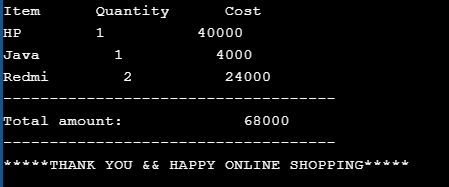Video Output: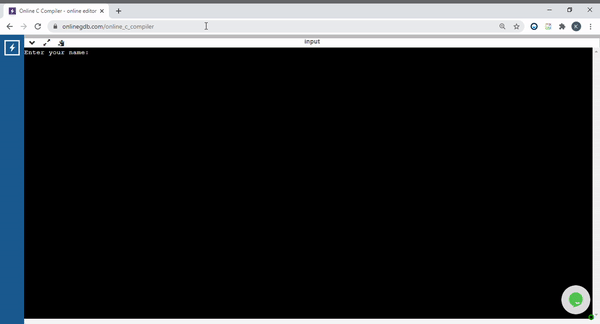Demonstration:

Step 1: Firstly, a map(say map<string, long double> items ) is constructed, which stores products with their costs. Construct another map (say map<string, long double>selected_items ), which is used to push the selected items with their quantity. Then initiate total_amount(which stores the total amount) to 0. Use flag and initiate to 0. In case if wrong input is given by the customer, then the flag changes to 1 and gets exited directly by printing items with their prices and then print the total amount.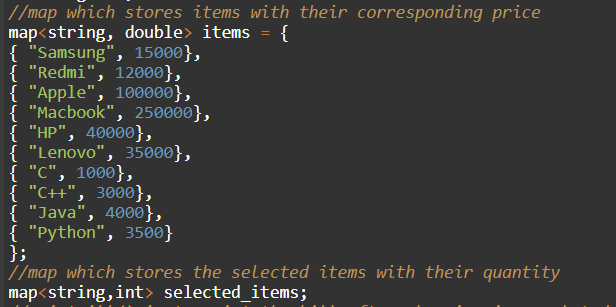Step 2: Ask for details For example- the name of the customer. In our code customerDetails() function is constructed for this purpose. toupper() is used for converting all the characters of a string into uppercase.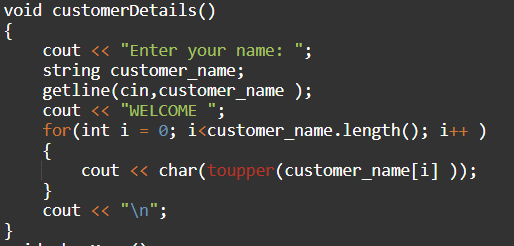Step 3: Display the menu to the user. showMenu() function is created for this purpose.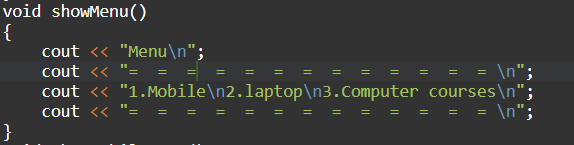Step 4: Ask the user whether he likes to continue. Here do-while loop is used, this loop continues till flag changes to 1. Whenever the flag changes to 1, it directly prints the bill.

1. If yes, he needs to enter Y/y then ask the user to input the respective number from the menu. If the wrong number is entered then the flag changes to 1.
1. If the input is valid, show the products of the selected type. Ask the user to input the respective number. If it is not valid, then the flag changes to 1.
2. As there are many products, a switch case is used, where the parameter is the number(respective number of the item) entered by user.
3. Now respective case gets executed. Firstly, ask the quantity and then ask the user if he is sure about the quantity entered. If he is not sure (or) if the quantity is not an integer, then he will be asked again till both conditions are satisfied.
4. If he is sure about the quantity of the product selected, then that product along with its quantity gets pushed into the selected_items map.
5. This process goes on till the flag gets changed to 1.
2. Else, he can type any other letter except Y/y. Then the flag changes to 1.

Below are some screenshots showing what will be the screen like, when the particular selection is done by the user-

• If mobile is selected: The below screenshot shows the Mobile Menu: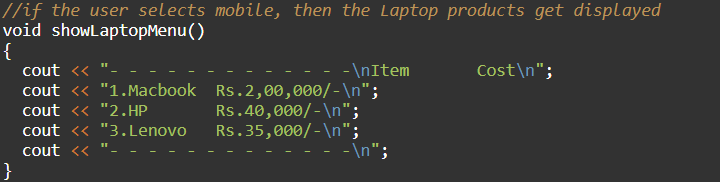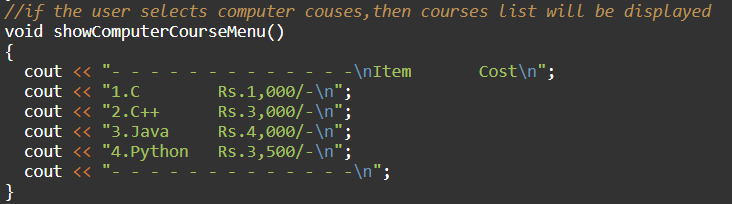My Personal Notes arrow_drop_up